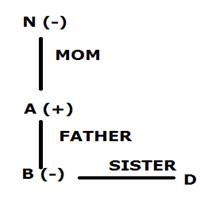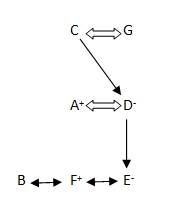## Blood Relations – Practice set 1

1. If P + Q means P is the mother of Q; P – Q means P is the brother Q; P % Q means P is the father of Q and P x Q means P is the sister of Q, which of the following shows that P is the maternal uncle of Q?

a) Q – N + M x P

b) P + S x N – Q

c) P – M + N x Q

d) Q – S % P

e) None of these

c) P – M + N x Q

C

Explanation

c

P – M → P is the brother of M

M + N → M is the mother of N

N x Q → N is the sister of Q

Therefore, P is the maternal uncle of Q.

2. If ‘P + Q’ means ‘P is father of Q’; ‘P % Q’ means ‘P is mother of Q’; ‘P & Q’ means ‘P is sister of Q’, then how is D related to N in N % A + B & D ?

a) Nephew

b) Grandson

c) Grand daughter

e) None of these

D

Explanation

d3. Pointing to a photograph, a man said, “I have no brother or sister but that man’s father is my father’s son” Who’s Photograph was it?

a) His own

b) His son’s

c) His father’s

d) His nephews

e) None of these

b) His son’s

B

Explanation

b

Since the narrator has no brother, his father’s son is he himself. So, the man who is talking is the father of the man in the photograph or the man in the photograph is his son.

4. E is brother of A. M is brother of A. H is father of M. T is wife of H. How is A related to T?

a) Son

b) Daughter

c) Son or Daughter

e) None of these

c) Son or Daughter

C

Explanation

c

As per question, H and T are Father and Mother

E is son, M is son and A is either son or daughter to T.

5. Navya is the only daughter of Mahesh’s sister Shalini’s brother. How is Navya related to Mahesh?

a) Daughter

b) Niece

c) Cousin

d) Niece or Daughter

d) Niece or Daughter

D

Explanation

d

As per the given data,

=>Navya = the only daughter of Mahesh’s sister Shalini’s brother

= The only daughter of Mahesh brother or Mahesh. So,

= Mahesh’s daughter/niece

D.6-7) Study the following information carefully and answer the question given below

i) ‘P×Q’ means ‘P’ is sister of ‘Q’

ii) ‘P+Q’ means ‘P is mother of Q’

iii) ‘P-Q’ means ‘P is father of Q’

iv) ‘P ÷Q’ means ‘P is brother of Q’

6. Which of the following represents ‘M is nephew of R’?

a) M ÷ T – R

b) R ÷ T –M

c) R × T + M × J

d) R ÷ T – M ÷ J

e) None of these

d) R ÷ T – M ÷ J

D

7. Which of the following represents ‘W is grandfather of H’?

a) W + T – H

b) W÷T-H

c) W × T + H

d) W ÷ T + H

e) None of these

e) None of these

E

8. Pointing to a person, Arun said to Sarika, “His mother is the only daughter of your father.” How is Sarika related to that person?

a) Aunt

b) Daughter

c) Mother-in-law

d) Mother

e) Sister

d) Mother

D

Explanation

d) Mother

Sarika’s father’s only daughter means Sarika herself, so Sarika is mother to that person (pointed to by His)

9. Which of the following means that M is grandmother of N, if ‘P \$ Q’ means P is father of Q’, ‘P*Q’ means P is mother of Q, ‘P @ Q’ means P is wife of Q’?

a) M * R \$ T @ N

b) M * R @ T @ N

c) M * T \$ N \$ R

d) M * T \$ R @ N

e) None of these

c) M * T \$ N \$ R

C

Explanation

c) M * T \$ N \$ R

Check all options
C) = M is mother of T, T father of N

Study the following information carefully and answer the questions given below:

In a family there are 7 persons and there are 2 married couples in this family

A is the father of B

E is the daughter of D and grand Daughter of C.

E is the sister of F, who is brother of B.

(I) How many female members are there?

a) 2

b) 3

c) 1

d) None of the above

e) Can’t be determined

e) Can’t be determined

E

Explanation

(E)(II) How many male members are there?

a) 2

b) 3

c) 4

d) Can’t be determined

e) None of the above

d) Can’t be determined

D

Explanation

(D)(III) Who is the daughter in law of the family

a) D

b) G

c) C

d) B

e) C